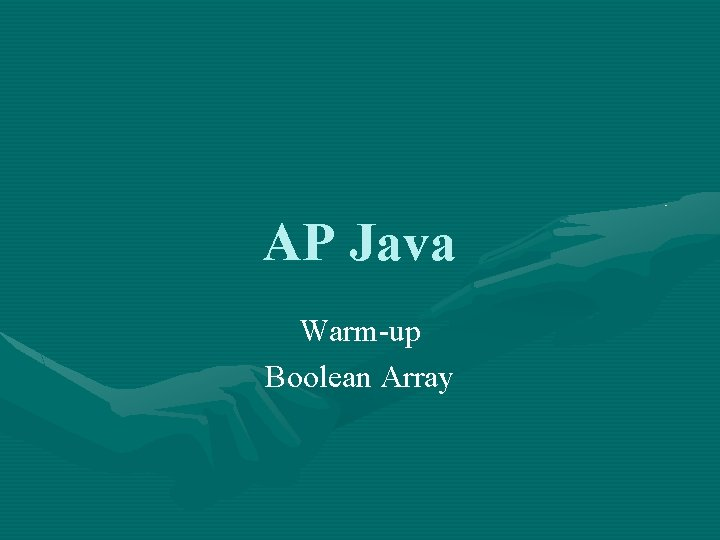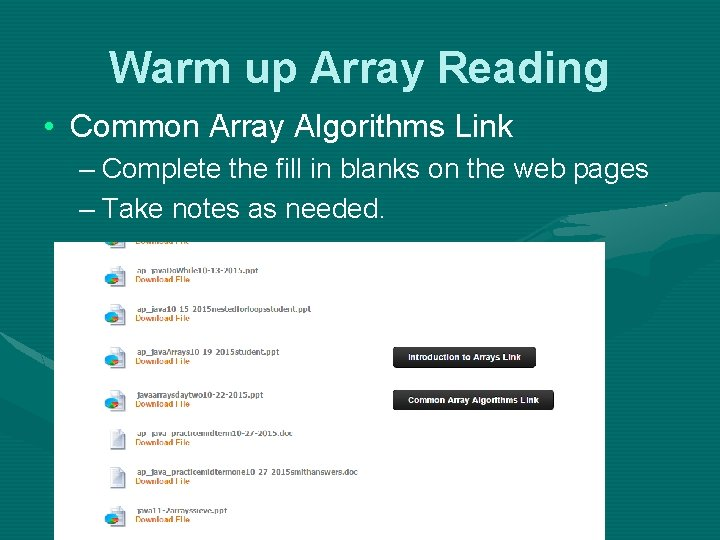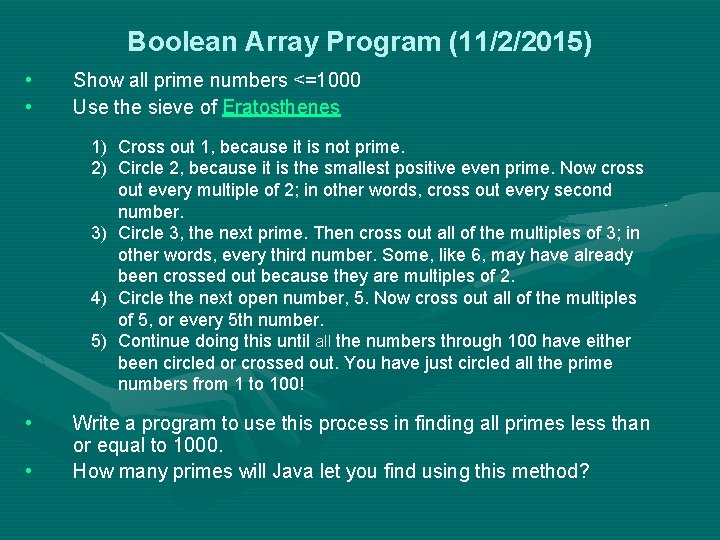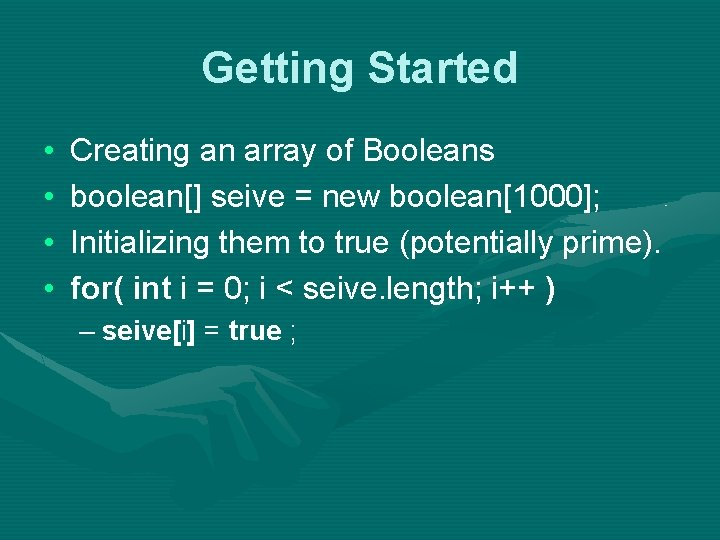# AP Java Warmup Boolean Array class Exercise 5

• Slides: 5AP Java Warm-up Boolean Arrayclass Exercise 5 { public static void main ( String[] args ) { int[] val = {0, 1, 2, 3}; int temp; System. out. println( "Original Array: " + val + " " + val + " " + val + " " + val ); // write the code to reverse the order of the numbers in the array System. out. println( "Reversed Array: " + val + " " + val + " " + val + " " + val ); } }Warm up Array Reading • Common Array Algorithms Link – Complete the fill in blanks on the web pages – Take notes as needed.Boolean Array Program (11/2/2015) • • Show all prime numbers <=1000 Use the sieve of Eratosthenes 1) Cross out 1, because it is not prime. 2) Circle 2, because it is the smallest positive even prime. Now cross out every multiple of 2; in other words, cross out every second number. 3) Circle 3, the next prime. Then cross out all of the multiples of 3; in other words, every third number. Some, like 6, may have already been crossed out because they are multiples of 2. 4) Circle the next open number, 5. Now cross out all of the multiples of 5, or every 5 th number. 5) Continue doing this until all the numbers through 100 have either been circled or crossed out. You have just circled all the prime numbers from 1 to 100! • • Write a program to use this process in finding all primes less than or equal to 1000. How many primes will Java let you find using this method?Getting Started • • Creating an array of Booleans boolean[] seive = new boolean; Initializing them to true (potentially prime). for( int i = 0; i < seive. length; i++ ) – seive[i] = true ;